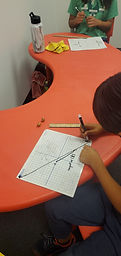Mr. Jake

Target 1​

Lesson Type:

Continuation

Algebra

:

Variable

Write a linear equation in slope-intercept form.

1:

Find the slope of a line on a graph.

2:

Define the slope as a rate of change.

3:

Use rise/run as a ratio to calculate the slope.

4:

Define the type of slope depicted in a linear equation as positive, negative, zero, and undefined.

7th

Vocabulary:

Slope, rise, run, point

Activities:

Determined the slope of a line using rise over run and the formula y2 - y1 / x2 - x1

Given two points, found the slope of a lineHome Exploration

Guiding Questions:Absent Students:

Target 2

:

2:

Use a protractor and understanding of percentages to construct pie graphs from given data.

3:

Analyze data presented in pie graphs to answer real-world questions.

7th

Vocabulary:

Pie chart, graph chart, Percent, degree, protractor

Activities:

Gathered data and created pie charts to represent the information

Used protractor and arithmetic to make precise pie chartsHome Exploration

Guiding Questions:Target 3

:

Vocabulary:

Activities:Home Exploration